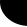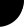HOME Electronics Directory Articles/ Tutorials eBooks About Us FORUM Links Contact UsVerilog Tutorial: Harsha Perla

if-else Statements

if statements allows the tool to decide a statement is to be executed or not, depending on the conditions specified. General syntax is as follows:

if( condition )
statement;

If the condition or conditional expression is true, then statement will be executed, otherwise not.

Consider the example

if( hold == 0 )
counter = counter + 1;

If reset is not zero, counter will be incremented.

else statement can be used with if optionally. If condition specified in if statement is false, statement after else will be executed. See this example, if reset is nonzero counter will become zero, othewise it will be incremented.

if( reset )
counter = 0;
else

counter = counter + 1;

If there are more than one statements within an if block, we can combine them using begin -- end. We can also nest if-else statements as in these examples.

if( reset )
begin
counter <= 0;
over_flow <= 0;
end
else if ( counter == 15 )
begin
counter <= 0;
over_flow <= 1;
end
else
begin

counter <= counter + 1;
over_flow <= 0;

end

if-else statements should be used inside initial or always blocks. Generally if-else statements generates multiplexers while synthesizing.

Here is a full Verilog code example using if else statements. This is a adder/ subtracter with 'addnsub' signal to control addition and subtraction.

 ```module addsub (a, b, addnsub, result); input[7:0] a; input[7:0] b; input addnsub; output[8:0] result; reg[8:0] result; always @(a or b or addnsub) begin if (addnsub) result = a + b; else result = a - b; end endmodule```

If addnsub is true( nonzero ), result will be a+b, otherwise result will be a-b.

Prev. : Blocking and nonblocking assignments